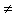# Pythagorean Triples and Perfect Numbers

One of the rather frequent quotes touching on the nature of mathematics is due to the famous Bertrand Russell:

... mathematics may be defined as the subject in which we never know what we are talking about, nor whether what we are saying is true.

Outside of the profession, the quote is mostly misunderstood to be derogatory of mathematics. (In an online poll, this is the second least picked out characterization of mathematics.) But, as John Paulos has observed in his influential book, Beyond Numeracy, the quote does give a succinct, albeit overstated, summary of the formal axiomatic approach to mathematics. Indeed much of mathematics consists of implications that deal with objects of an entirely abstract nature. Even the simplest of the mathematical objects, say natural numbers, are abstract entities that one does not meet in a real world. Ron Aharoni in his recent Arithmetic for Parents suggests a surprise request in a first lesson on denominations: "Give me two," which underscores the abstractedness of the number.

A rich history of perfect numbers has been recently supplemented by an implication of the sort that B. Russell had in mind.

### A Perfect Hypotenuse

A Pythagorean triple is an ordered triple (a, b, c) of positive integers satisfying a² + b² = c². The number c is called the hypotenuse of the Pythagorean triple.

1. Prove that an even perfect number cannot be the hypotenuse of a Pythagorean triple.
2. Prove that if there is an odd perfect number, then it is the hypotenuse of a Pythagorean triple.

(A natural number is perfect if it is equal to the sum of all its divisors excluding itself.)

Observe the second part of the problem. While the even perfect numbers have been an object of study from the time of Euclid, no odd perfect number has ever been found. No one was able to prove that they do not exist either; there are none below 10300 and although there are serious doubts there are any beyond this bound, a group of enthusiastic devotees is currently working on raising it to 10600.

The problem is in the spirit of many results concerning odd perfect numbers, the first of which is probably due to Frenicle (1657), see [Dickson, p. 14] followed by L. Euler's [Dickson, p. 19]. Euler showed that any odd perfect number is bound to be in the form r4λ + 1P², where r is a prime of the form 4n + 1.

But to return to the problem, an even perfect number n must be of the form 2k-1(2k - 1) with 2k - 1 prime. In addition, a number is the hypotenuse of a Pythagorean triple if and only if it is a multiple of a number of the form t = r² + s² where r and s are relatively prime positive integers and have opposite parity. Thus, t = 1 (mod 4). Because 2k - 1 = 3 (mod 4), it follows that the number n cannot be a multiple of such a number t. This answers part (a) of the problem.

By a result of Euler, an odd perfect number must be of the form n = pαm² with p prime and p = α = 1 (mod 4). By a result of Fermat [Hardy, p. 299], n can be represented as the sum of two squares if and only if every prime factor congruent to 3 modulo 4 in the prime factorization of n occurs with an even exponent. It follows that if n is an odd perfect number, then n = x² + y² for some positive integers x and y, xy. Because (x² - y²)² + (2xy)² = n², we see that n is the hypotenuse of a Pythagorean triple.As a matter of fact, Descartes believed in the existence of odd perfect numbers. He came very close to finding one with the following example [Klee and Wagon, p. 180]. Let

N = 22021·3²·7²·11²·13².

Indeed, since for prime numbers p, σ(p) = p + 1 and, more generally, for a power of a prime, σ(pn) = 1 + p + ... + pn,

∑(N) = (22021 + 1)·(1 + 3 + 3²)·(1 + 7 + 7²)·(1 + 11 + 11²)·(1 + 13 + 13²) = 2N.

Here σ(N) is the sum of all divisors of N, including 1 and N. In terms of σ, N is perfect iff σ(N) = 2N, as in Descartes' example. However, something is wrong here, but what?

1. R. Aharoni, Arithmetic for Parents, Sumizdat, 2007.
2. L. E. Dickson, History of the Theory of Numbers, Vol. 1: Divisibility and Primality, Dover, 2005.
3. R. L Doucette, Solution to problem 1737 proposed by M. Z. Spivey, Mathematics Magazine, v 80, n 1 (February 2007).
4. G. H. Hardy, E. M. Wright, An Introduction to the Theory of Numbers, Fifth Edition, Oxford Science Publications, 1996
5. V. Klee, S. Wagon, Old and New Unsolved Problems in Plane Geometry and Number Theory, MAA, 1991.
6. J. A. Paulos, Beyond Numeracy, Vintage Books, 1992.Pythagorean triples